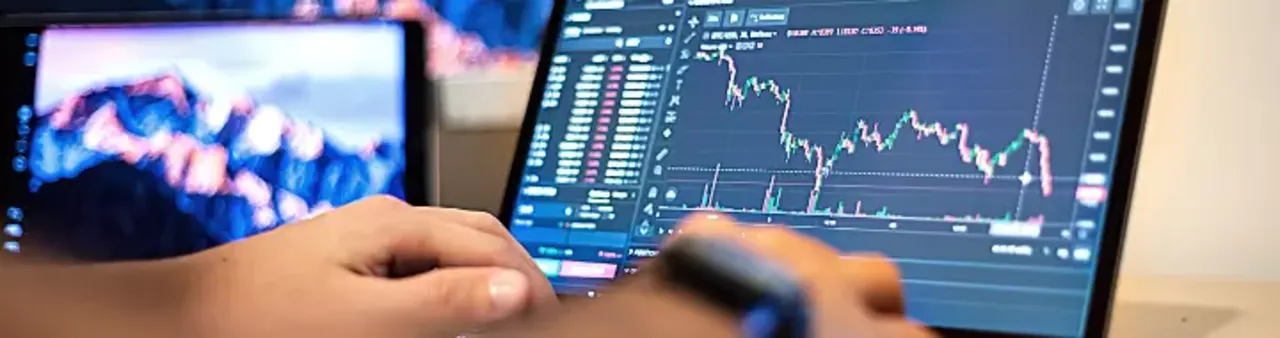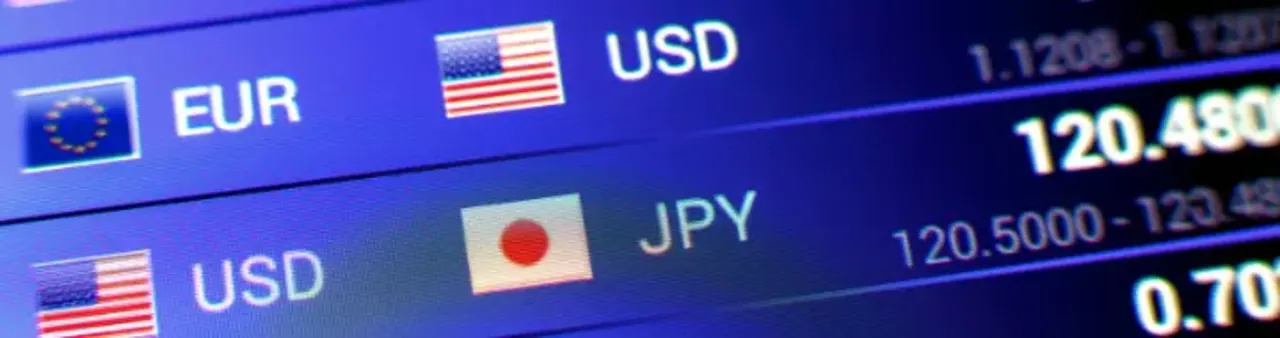# Dollar Value of Pips

Daksh Murkute | | |

You’ll hear lots of traders claiming that they profited 50 pips, 100 pips, or 500 pips.

But, how much does it mean in dollar terms? How much did they actually profit? A good question to ask right?

Knowing the dollar value of pips is very important in forex trading and in this blog post, I’ll show you just that.

So read this blog post till the end to know how much each pip that you’ll profit is worth and how to calculate it.

### Contents

Basics of pips

Purpose of pips

Why do you need to know the dollar value of pips?

How to calculate the dollar value of each pip?

Pips are the smallest unit of price change in forex and the value of each pip will differ from currency pair to currency pair. If you're a trader then you need to know how to calculate them before you trade as it has a lot of importance.

## Basics of pipsIn forex trading, each price movement is represented by pips.

Pip stands for Price in Percentage and it is the minimum change in price that can take place in the currency pairs.

Whenever you see the price quote of currency pairs, you’ll see that it is in decimals, for example, the price of the EURUSD currency pair is 1.1377.

In this, the last 7 that is at the 4th place after the decimal, is the pip.

All currency pairs follow this system of pips except the JPY currency.

Currency pairs with the JPY as the quote currency will have the pips at the 2nd place after the decimal.

## Purpose of pipsThe purpose of pips is simple, it is to show the price change.

If the price of the EURUSD currency pair was 1.1377 yesterday and today it is 1.1387, what does it mean?

It means that the price increased by 10 pips.

I’m sure you know that currencies are traded in pairs on the forex market.

And this would make it a bit difficult to track the price change, that’s why we have pips in place.

Tracking price change becomes difficult as we can different currencies as the quote currency.

Now, it is because of this thing that the dollar value of pips is different and read ahead to know more about it.

## Why do you need to know the dollar value of pips?You need to know how much each pip is worth for two reasons, first, to know how much you have earned in total after you have profited some pips, and second, for risk management.

As I said earlier, you can profit 100s of pips but that’s not enough right, you need to know exactly how much are those pips worth in total.

Coming to the second thing, knowing the value of each pip helps in managing risk. How is that?

One of the biggest aspects of risk management is position sizing and you need to calculate this before you take any trade.

The formula for position sizing is this,

Position size = Total amount being risked / (Total pips being risk x Dollar value of each pip)

Do you see that? You cannot calculate position size without knowing how much each pip is worth in the currency pair that you are trading.

That’s why it is very important to know how to calculate the dollar value of each pip and I’m going to show you how to do that right away.

## How to calculate the dollar value of each pip?

I’m sure by now you know what exactly pips are, their purpose, and also why exactly you need to know how much each pip is worth.

Now, when it comes to calculating the dollar value of each pip, there are some things that you need to keep in mind.

You need to know which currency is the quote currency and what kind of lot are you trading.

### Account currency same as the quote currencyIf the quote currency is the USD and your account is also in USD, then each pip will be worth \$1, if you are trading the mini lots.

With standard lots, each pip will be worth \$10 and on the other hand, with micro-lots, each pip will be worth \$0.1

Now say that you are following our blog on order block and order flow to trade currency pairs like EURUSD, GBPUSD, AUDUSD, NZDUSD, etc. with mini lots then, each pip that you profit will fetch you \$1.

On the other hand, if you lose a pip, you will incur a \$1.

Likewise, if the quote currency is GBP and your account is also in GBP, then each pip’s value will be GBP 1, GBP10, and GBP 0.1 for a mini lot, standard lot, and micro lot respectively.

The profit and loss of each pip will also reflect the same as I’ve shown you above.

But, what if the account currency is not the same as the quote currency? Then how much will a pip be worth?

### Account currency is different from quote currencyThis is where things can become a little bit tricky when it comes to calculating the value of each pip.

Don’t worry, in this you don’t have to do anything too big, it’s just a formula that you need to keep in mind.

Let’s say that you are trading with an account that is in USD and the currency pair that you are looking to trade is EURGBP.

What’s the quote currency here? Yes, that’s right, it’s the GBP.

Now if you want to know how much each pip will be worth in this currency pair, you first need to find out the price of the USDGBP currency pair.

Let’s say that at the moment this currency pair is trading at 0.7435.

Divide 10 by this figure that is 0.7435, the answer is 13.45. This is how much each pip will be worth in dollar terms if you are trading with a USD account and are using standard lots.

For mini lots, the value of each pip will be \$1.345 and for micro-lots, it will be \$0.1345.

This was just for an account in USD, but you can apply this same concept to find out how much each pip will be worth if you’re using an account in a different currency.